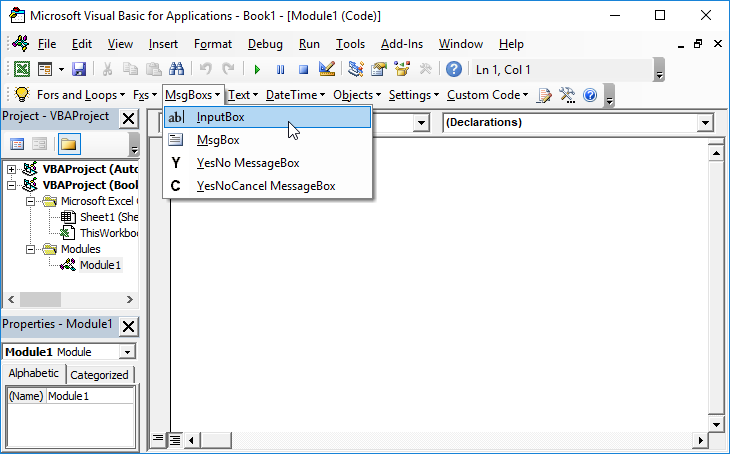# VBA InputBox – Get Input from a User

This tutorial will demonstrate how to get input from a user with the VBA Input Box.

The VBA Input Box allows us to prompt the user to input information.  The information can then be used in our VBA Code or in an Excel worksheet.

## The VBA Input Box with a Variable

We can declare a variable in our VBA code which then stores the information that is typed into the Input Box.  We can then use this variable in our code.

Depending on the type of information we want returned from the input box, we can declare a specific type of variable to either return text (a string or variant variable) or to return a number (an integer, long, double or single variable).

### The String Variable with an Input Box

Here is how you can create an InputBox where the data is returned into a string variable.

``````Dim strInput as String

strInput = InputBox("This is my InputBox", "MyInputTitle", "Enter your input text HERE")``````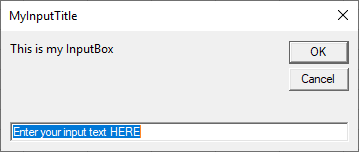Notice first we declare a variable. This variable will be assigned the value that the user enters.  We used variable type String in order for the Input box to accept text strings (which includes any alpha numeric characters).

### The Number Variable with an Input Box

If you declare a number variable, you will need to enter a number only into the input box.

``````Dim iInput As Integer
iInput = InputBox("Please enter a number", "Create Invoice Number", 1)``````

First we declare the number variable as an integer variable.   We can then also put a default value of 1 in the input box.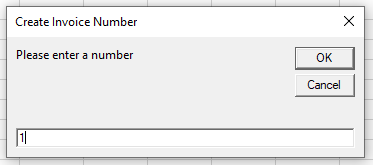If we were to enter a string instead of a number and then click on OK, we would get a Type Mismatch Error as a number variable does not accept a string.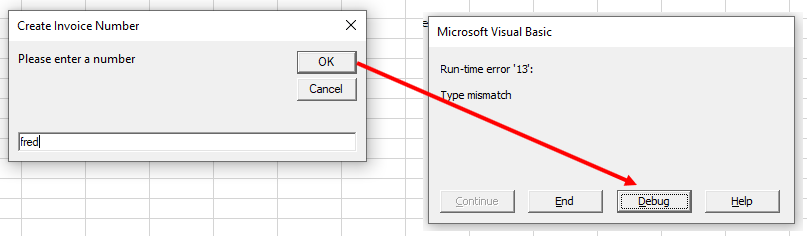## Get Input from a User

Here is another example using the most popular features of an InputBox.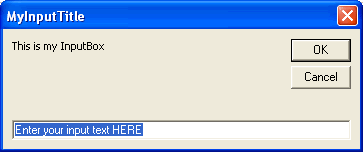The following code does three things:

1. Prompts for input with an InputBox and assigns it to a variable

2. Verifies input, otherwise exits the sub

3. Returns the input in a message box

``````Public Sub MyInputBox()

Dim MyInput As String
MyInput = InputBox("This is my InputBox", "MyInputTitle", "Enter your input text HERE")

If MyInput = "Enter your input text HERE" Or MyInput = "" Then
Exit Sub
End If

MsgBox "The text from MyInputBox is " & MyInput

End Sub``````

## Returning the input to an Excel Sheet

You can return the input that you type into an input box into a specific cell in your Excel sheet.

``Range("P1") = InputBox("Please type in your name", "Enter Name", "Enter name HERE")``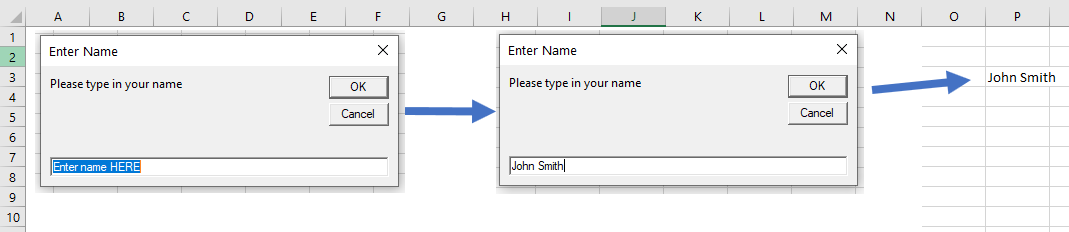We can also return input data to an Excel Sheet using a variable.

``````Sub EnterNumber()
On Error Resume Next
Dim dblAmount As Double
dblAmount = InputBox("Please enter the required amount", "Enter Amount")
If dblAmount <> 0 Then
Range("A1") = dblAmount
Else
MsgBox "You did not enter a number!"
End If
End Sub``````

In the example above, we are required to enter a number.  If we enter a number, then the variable dblAmount will put the number into the Excel sheet at cell A1.  However, if we do not enter a number, then a message box will tell us that we did not enter a number and nothing will be put into cell A1.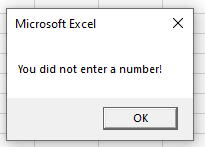## Using VBA InputBox in Access VBA

The VBA input box works exactly the same way in Access as it does in Excel when returning user input in the form of a message box.

However, if you wish to return the user input to the database, you would need to use the Recordset object rather than the Range object as used in Excel.

``````Sub EnterInvoiceNumber()
Dim dbs As Database
Dim rst As Recordset
Set dbs = CurrentDb
Set rst = dbs.OpenRecordset("tblMatters", dbOpenDynaset)
With rst
!InvNo = InputBox("Please enter the Invoice Number", "INVOICE NUMBER GENERATION", 1)
.Update
End With
rst.Close
Set rst = Nothing
Set dbs = Nothing
End Sub``````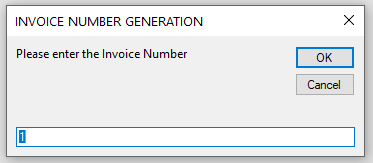Stop searching for VBA code online. Learn more about AutoMacro – A VBA Code Builder that allows beginners to code procedures from scratch with minimal coding knowledge and with many time-saving features for all users!